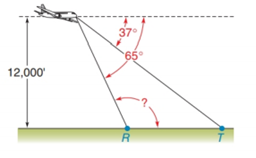Chapter 2.4, Problem 36E### Elementary Geometry for College St...

6th Edition
Daniel C. Alexander + 1 other
ISBN: 9781285195698

#### Solutions

Chapter
Section### Elementary Geometry for College St...

6th Edition
Daniel C. Alexander + 1 other
ISBN: 9781285195698
Textbook Problem
1 views

# An airplane has leveled off (is flying horizontally) at an altitude of 12, 000 feet. Its pilot can see each of two farmhouses at points R and T in front of the plane. With angle measures as indicated, find m ∠ RTo determine

To find:

The value of mR.

Explanation

Given:

The airplane is at an altitude of 12, 000 feet.

Figure (1)

Approach:

The sum of the interior angles on the same side of the transversal which cut by two parallel lines is equal to 180°.

Calculation:

R and 65° are two angles on the same side of the transversal as shown in figure (1).

### Still sussing out bartleby?

Check out a sample textbook solution.

See a sample solution

#### The Solution to Your Study Problems

Bartleby provides explanations to thousands of textbook problems written by our experts, many with advanced degrees!

Get Started

#### The second partial derivative zyx of the function z=x2y+yex2 at the point (1,2).

Mathematical Applications for the Management, Life, and Social Sciences

#### Sketch the graphs of the equations in Exercises 512. xy=x2+1

Finite Mathematics and Applied Calculus (MindTap Course List)

#### If f(x)=x2xx1andg(x)=x is it true that f = g?

Single Variable Calculus: Early Transcendentals

#### True or False: f(x) = x2 is decreasing for 10 x 1.

Study Guide for Stewart's Single Variable Calculus: Early Transcendentals, 8th